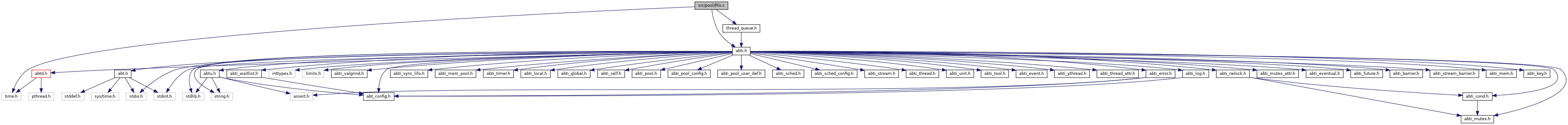ARGOBOTS  1227c643f7a7f974f1f1778a9ffebd29d7dafecf
fifo.c File Reference
`#include "abti.h"`
`#include <time.h>`
Include dependency graph for fifo.c:Go to the source code of this file.

struct  data

## Typedefs

typedef struct data data_t

## Functions

static int pool_init (ABT_pool pool, ABT_pool_config config)

static int pool_free (ABT_pool pool)

static size_t pool_get_size (ABT_pool pool)

static void pool_push_shared (ABT_pool pool, ABT_unit unit)

static void pool_push_private (ABT_pool pool, ABT_unit unit)

static ABT_unit pool_pop_shared (ABT_pool pool)

static ABT_unit pool_pop_private (ABT_pool pool)

static ABT_unit pool_pop_wait (ABT_pool pool, double time_secs)

static ABT_unit pool_pop_timedwait (ABT_pool pool, double abstime_secs)

static int pool_remove_shared (ABT_pool pool, ABT_unit unit)

static int pool_remove_private (ABT_pool pool, ABT_unit unit)

static int pool_print_all (ABT_pool pool, void *arg, void(*print_fn)(void *, ABT_unit))

static ABT_unit_type unit_get_type (ABT_unit unit)

static ABT_bool unit_is_in_pool (ABT_unit unit)

static void unit_free (ABT_unit *unit)

static data_tpool_get_data_ptr (void *p_data)

ABTU_ret_err int ABTI_pool_get_fifo_def (ABT_pool_access access, ABT_pool_def *p_def)

## Typedef Documentation

 typedef struct data data_t

Definition at line 39 of file fifo.c.

## Function Documentation

 ABTU_ret_err int ABTI_pool_get_fifo_def ( ABT_pool_access access, ABT_pool_def * p_def )

Definition at line 47 of file fifo.c.

Referenced by ABTI_pool_create_basic().

 static int pool_free ( ABT_pool pool )
static

Definition at line 127 of file fifo.c.

Referenced by ABTI_pool_get_fifo_def().

 static data_t* pool_get_data_ptr ( void * p_data )
inlinestatic

Definition at line 41 of file fifo.c.

 static size_t pool_get_size ( ABT_pool pool )
static

Definition at line 138 of file fifo.c.

Referenced by ABTI_pool_get_fifo_def().

 static int pool_init ( ABT_pool pool, ABT_pool_config config )
static

Definition at line 100 of file fifo.c.

Referenced by ABTI_pool_get_fifo_def().

 static ABT_unit pool_pop_private ( ABT_pool pool )
static

Definition at line 318 of file fifo.c.

Referenced by ABTI_pool_get_fifo_def().

 static ABT_unit pool_pop_shared ( ABT_pool pool )
static

Definition at line 287 of file fifo.c.

Referenced by ABTI_pool_get_fifo_def().

 static ABT_unit pool_pop_timedwait ( ABT_pool pool, double abstime_secs )
static

Definition at line 245 of file fifo.c.

Referenced by ABTI_pool_get_fifo_def().

 static ABT_unit pool_pop_wait ( ABT_pool pool, double time_secs )
static

Definition at line 197 of file fifo.c.

Referenced by ABTI_pool_get_fifo_def().

 static int pool_print_all ( ABT_pool pool, void * arg, void(*)(void *, ABT_unit) print_fn )
static

Definition at line 414 of file fifo.c.

Referenced by ABTI_pool_get_fifo_def().

 static void pool_push_private ( ABT_pool pool, ABT_unit unit )
static

Definition at line 172 of file fifo.c.

Referenced by ABTI_pool_get_fifo_def().

 static void pool_push_shared ( ABT_pool pool, ABT_unit unit )
static

Definition at line 145 of file fifo.c.

Referenced by ABTI_pool_get_fifo_def().

 static int pool_remove_private ( ABT_pool pool, ABT_unit unit )
static

Definition at line 382 of file fifo.c.

Referenced by ABTI_pool_get_fifo_def().

 static int pool_remove_shared ( ABT_pool pool, ABT_unit unit )
static

Definition at line 347 of file fifo.c.

Referenced by ABTI_pool_get_fifo_def().

static

Definition at line 494 of file fifo.c.

Referenced by ABTI_pool_get_fifo_def().

static

Definition at line 482 of file fifo.c.

Referenced by ABTI_pool_get_fifo_def().

 static void unit_free ( ABT_unit * unit )
static

Definition at line 505 of file fifo.c.

Referenced by ABTI_pool_get_fifo_def().

static

Definition at line 463 of file fifo.c.

Referenced by ABTI_pool_get_fifo_def().

static

Definition at line 450 of file fifo.c.

Referenced by ABTI_pool_get_fifo_def().

 static ABT_unit_type unit_get_type ( ABT_unit unit )
static

Definition at line 444 of file fifo.c.

Referenced by ABTI_pool_get_fifo_def().

 static ABT_bool unit_is_in_pool ( ABT_unit unit )
static

Definition at line 475 of file fifo.c.

Referenced by ABTI_pool_get_fifo_def().